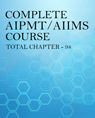Click to Chat

1800-1023-196

+91-120-4616500

CART 0

• 0

MY CART (5)

Use Coupon: CART20 and get 20% off on all online Study Material

ITEM
DETAILS
MRP
DISCOUNT
FINAL PRICE
Total Price: Rs.

There are no items in this cart.
Continue Shopping
`        in the adjoining figure the area enclosed between the concentric circles is 770 cm2 if the radius of the outer circle is 21 cm calculate the radius of inner. circle`
2 years ago

```							Please enclose the proper supporting document
```
2 years ago
```							Given :Radius of outer circle = 21cm.Area between inner & outer circle = 770cm2. Area of outer circle = r2                                     = 22/7 x 212                                     = 1386cm2. Area of innercircle = 1386-770                              = 616cm2. r2 = 616      r2 = 616 x 7/22      r  =  14cm. Therefore radius of innercircle = 14cm.
```
2 years ago
```							here we havethe radius of outer circle= 21cmarea between the concentric circle =7702 R2 = 22/7*21*21           =1386cm r2  =1386cm -770cm          = 616cmso,radius of inner circle r2 = 22/7*r2616 =22/7*r2616*7/22 = r2196 = r2142 = r214 = rhence radius = 14 cm
```
one year ago
Think You Can Provide A Better Answer ?

## Other Related Questions on AIIMS MBBS

View all Questions »### Course Features

• 731 Video Lectures
• Revision Notes
• Previous Year Papers
• Mind Map
• Study Planner
• NCERT Solutions
• Discussion Forum
• Test paper with Video Solution### Course Features

• 728 Video Lectures
• Revision Notes
• Test paper with Video Solution
• Mind Map
• Study Planner
• NCERT Solutions
• Discussion Forum
• Previous Year Exam Questions